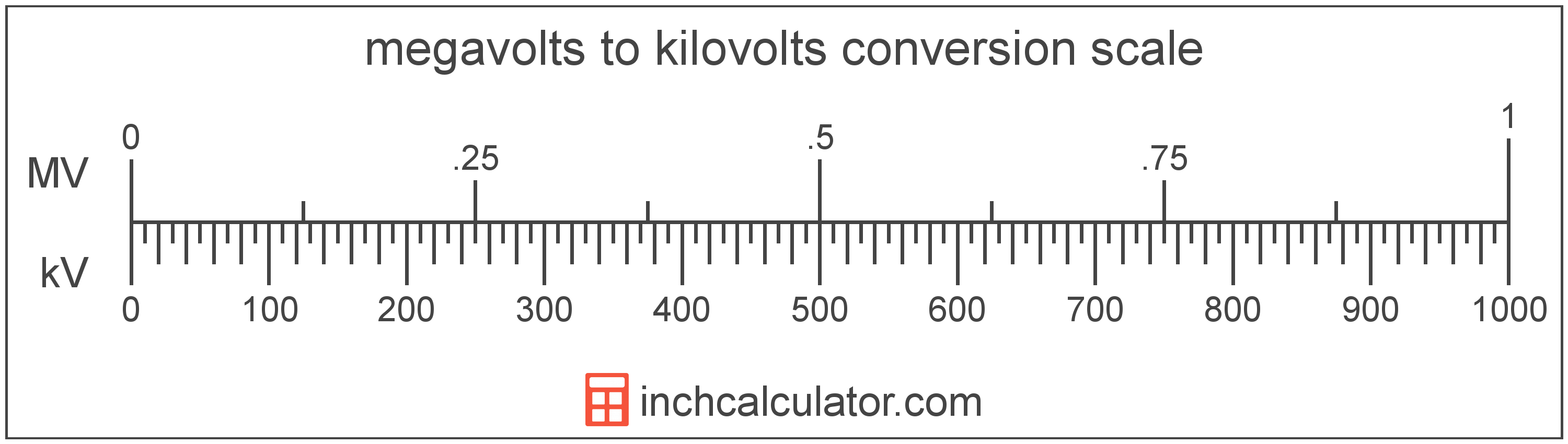# Kilovolts to Megavolts Converter

Enter the voltage in kilovolts below to get the value converted to megavolts.

Results in Megavolts:1 kV = 0.001 MV

Do you want to convert megavolts to kilovolts?

## How to Convert Kilovolts to Megavolts

To convert a measurement in kilovolts to a measurement in megavolts, divide the voltage by the following conversion ratio: 1,000 kilovolts/megavolt.

Since one megavolt is equal to 1,000 kilovolts, you can use this simple formula to convert:

megavolts = kilovolts ÷ 1,000

The voltage in megavolts is equal to the voltage in kilovolts divided by 1,000.

For example, here's how to convert 5,000 kilovolts to megavolts using the formula above.
megavolts = (5,000 kV ÷ 1,000) = 5 MV## What Is a Kilovolt?

One kilovolt is equal to 1,000 volts, which are the potential difference that would move one ampere of current against one ohm of resistance.

The kilovolt is a multiple of the volt, which is the SI derived unit for voltage. In the metric system, "kilo" is the prefix for thousands, or 103. Kilovolts can be abbreviated as kV; for example, 1 kilovolt can be written as 1 kV.

## What Is a Megavolt?

One megavolt is equal to 1,000,000 volts, which are the potential difference that would move one ampere of current against one ohm of resistance.

The megavolt is a multiple of the volt, which is the SI derived unit for voltage. In the metric system, "mega" is the prefix for millions, or 106. Megavolts can be abbreviated as MV; for example, 1 megavolt can be written as 1 MV.

## Kilovolt to Megavolt Conversion Table

Table showing various kilovolt measurements converted to megavolts.
Kilovolts Megavolts
1 kV 0.001 MV
2 kV 0.002 MV
3 kV 0.003 MV
4 kV 0.004 MV
5 kV 0.005 MV
6 kV 0.006 MV
7 kV 0.007 MV
8 kV 0.008 MV
9 kV 0.009 MV
10 kV 0.01 MV
20 kV 0.02 MV
30 kV 0.03 MV
40 kV 0.04 MV
50 kV 0.05 MV
60 kV 0.06 MV
70 kV 0.07 MV
80 kV 0.08 MV
90 kV 0.09 MV
100 kV 0.1 MV
200 kV 0.2 MV
300 kV 0.3 MV
400 kV 0.4 MV
500 kV 0.5 MV
600 kV 0.6 MV
700 kV 0.7 MV
800 kV 0.8 MV
900 kV 0.9 MV
1,000 kV 1 MV